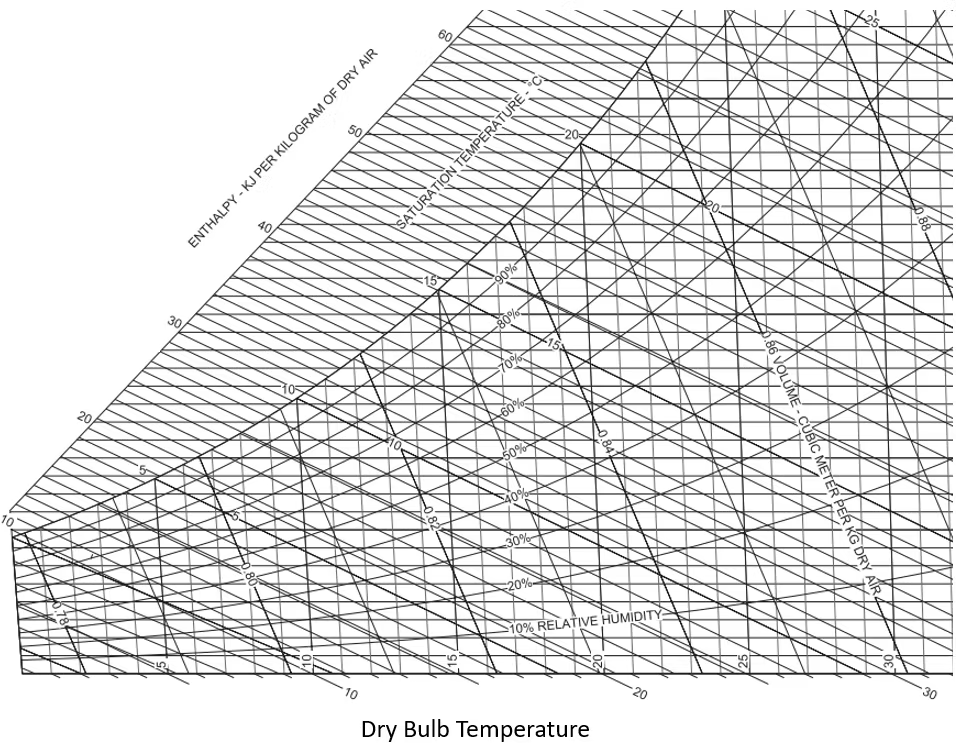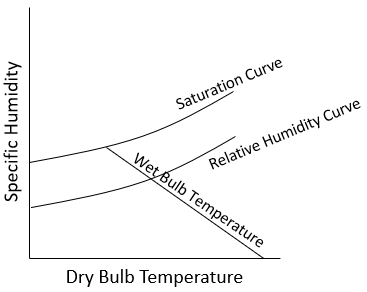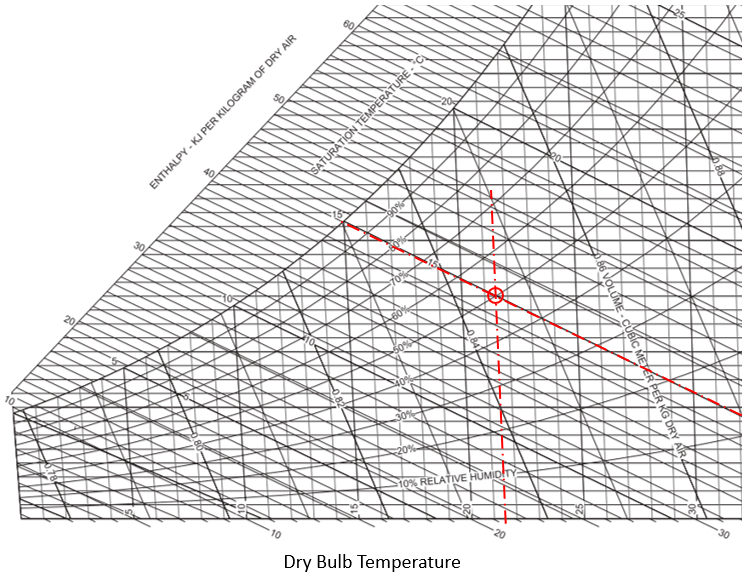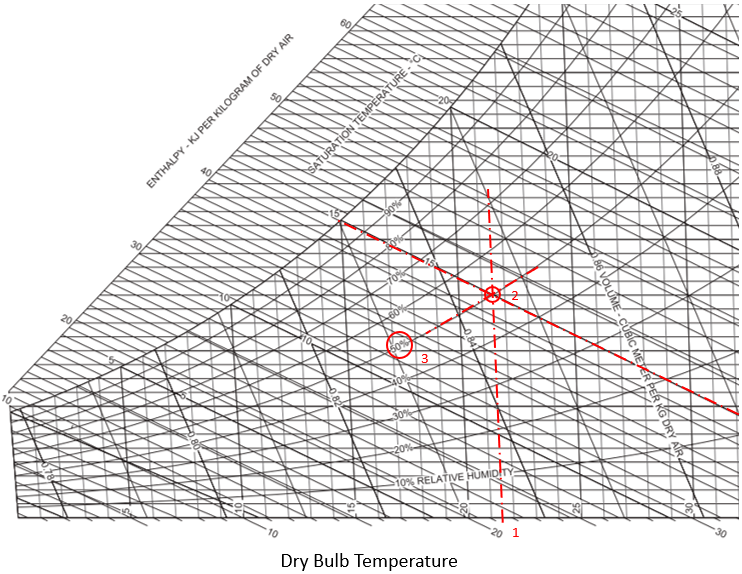## Relative Humidity

Find the relative humidity if the actual air temperature is 21.5°C and the wet-bulb temperature is 15°C.Hint
Properties of an air-water vapor mixture at a fixed pressure are given in graphical form on a psychrometric chart as provided in problem statement. When the system pressure is 1 atm, an ideal-gas mixture is assumed.Hint 2Properties of an air-water vapor mixture at a fixed pressure are given in graphical form on a psychrometric chart as provided in problem statement. When the system pressure is 1 atm, an ideal-gas mixture is assumed.In the psychrometric chart, let’s find where our 21.5°C dry bulb temperature (the temperature of air measured by a thermometer freely exposed to air) intersects with the 15°C wet-bulb temperature (2). The relative humidity curve that crosses this intersection is what we are looking for (3).The relative humidity is 50%.
50%